# Welcome to the jupyter_rfb docs!¶

The jupyter_rfb library provides a widget (an ipywidgets subclass) that can be used in the Jupyter notebook and in JupyterLab to realize a remote frame buffer.Images that are generated at the server are streamed to the client (Jupyter) where they are shown. Events (such as mouse interactions) are streamed in the other direction, where the server can react by generating new images.

This remote-frame-buffer approach can be an effective method for server-generated visualizations to be dispayed in Jupyter notebook/lab. For example visualization created by tools like vispy, datoviz or pygfx.

## Contents¶

### The jupyter_rfb guide¶

#### Installation¶

Install with pip:

```pip install -U jupyter_rfb
```

Or to install into a conda environment:

```conda install -c conda-forge jupyter-rfb
```

For better performance, also install `simplejpeg` or `pillow`.

If you plan to hack on this library, see the contributor guide for a dev installation and more.

#### Subclassing the widget¶

The provided `RemoteFrameBuffer` class cannot do much by itself, but it provides a basis for widgets that want to generate images at the server, and be informed of user events. The way to use `jupyter_rfb` is therefore to create a subclass and implement two specific methods.

The first method to implement is `.get_frame()`, which should return a uint8 numpy array. For example:

```class MyRemoteFrameBuffer(jupyter_rfb.RemoteFrameBuffer):

def get_frame(self):
return np.random.uniform(0, 255, (100,100)).astype(np.uint8)
```

The second method to implement is `.handle_event()`, which accepts an event object. This is where you can react to changes and user interactions. The most important one may be the resize event, so that you can match the array size to the region on screen. For example:

```class MyRemoteFrameBuffer(jupyter_rfb.RemoteFrameBuffer):

def handle_event(self, event):
event_type = event["event_type"]
if event_type == "resize":
self.logical_size = event["width"], event["height"]
self.pixel_ratio = event["pixel_ratio"]
elif event_type == "pointer_down":
pass  # ...
```

#### Logical vs physical pixels¶

The size of e.g. the resize event is expressed in logical pixels. This is a unit of measurement that changes as the user changes the browser zoom level.

By multiplying the logical size with the pixel-ratio, you obtain the physical size, which represents the actual pixels of the screen. With a zoom level of 100% the pixel-ratio is 1 on a regular display and 2 on a HiDPI display, although the actual values may also be affected by the OS’s zoom level.

#### Scheduling draws¶

The `.get_frame()` method is called automatically when a new draw is performed. There are cases when the widget knows that a redraw is (probably) required, such as when the widget is resized.

If you want to trigger a redraw (e.g. because certain state has changed in reaction to user interaction), you can call `.request_draw()` to schedule a new draw.

The scheduling of draws is done in such a way to avoid images being produced faster than the client can consume them - a process known as throttling. In more detail: the client sends a confirmation for each frame that it receives, and the server waits with producing a new frame until the client has confirmed receiving the nth latest frame. This mechanism causes the calls to `.get_frame()` to match the speed by which the frames can be communicated and displayed. This helps minimize the lag and optimize the FPS.

#### Event throttling¶

Events go from the client (browser) to the server (Python). Some of these are throttled so they are emitted a maximum number of times per second. This is to avoid spamming the communication channel and server process. The throttling applies to the resize, scroll, and pointer_move events.

#### Taking snapshots¶

In a notebook, the `.snapshot()` method can be used to create a picture of the current state of the widget. This image remains visible when the notebook is in off-line mode (e.g. in nbviewer). This functionality can be convenient if you’re using a notebook to tell a story, and you want to display a certain result that is still visible in off-line mode.

When a widget is first displayed, it automatically creates a snapshot, which is hidden by default, but becomes visible when the widget itself is not loaded. In other words, example notebooks have pretty pictures!

#### Exceptions and logging¶

The `.handle_event()` and `.get_frame()` methods are called from a Jupyter COM event and in an asyncio task, respectively. Under these circumstances, Jupyter Lab/Notebook may swallow exceptions as well as writes to stdout/stderr. See issue #35 for details. These are limitation of Jupyter, and we should expect these to be fixed/improved in the future.

In jupyter_rfb we take measures to make exceptions raised in either of these methods result in a traceback shown right above the widget. To ensure that calls to `print()` in these methods are also shown, use `self.print()` instead.

Note that any other streaming to stdout and stderr (e.g. logging) may not become visible anywhere.

#### Measuring statistics¶

The `RemoteFrameBuffer` class has a method `.get_stats()` that returns a dict with performance metrics:

```>>> w.reset_stats()  # start measuring
... interact or run a test
>>> w.get_stats()
{
...
}
```

#### Performance tips¶

The framerate that can be obtained depends on a number of factors:

• The size of a frame: smaller frames generally encode faster and result in smaller blobs, causing less strain on both CPU and IO.

• How many widgets are drawing simultaneously: they use the same communication channel.

• The `widget.quality` trait: lower quality results in faster encoding and smaller blobs.

• When using lossless images (`widget.quality == 100`), the entropy (information density) of a frame also matters, because for PNG, high entropy data takes longer to compress and results in larger blobs.

For more details about performance considerations in the implementation of `jupyter_rfb`, see issue #3.

### The jupyter_rfb reference¶

#### RemoteFrameBuffer class¶

class jupyter_rfb.RemoteFrameBuffer(**kwargs)

A widget implementing a remote frame buffer.

This is a subclass of ipywidgets.DOMWidget. To use this class, it should be subclassed, and its `.get_frame()` and `.handle_event()` methods should be implemented.

This widget has the following traits:

• css_width: the logical width of the frame as a CSS string. Default ‘500px’.

• css_height: the logical height of the frame as a CSS string. Default ‘300px’.

• resizable: whether the frame can be manually resized. Default True.

• quality: the quality of the JPEG encoding during interaction/animation as a number between 1 and 100. Default 80. Set to lower numbers for more performance on slow connections. Note that each interaction is ended with a lossless image (PNG). If set to 100 or if JPEG encoding isn’t possible (missing pillow or simplejpeg dependencies), then lossless PNGs will always be sent.

• max_buffered_frames: the number of frames that is allowed to be “in-flight”, i.e. sent, but not yet confirmed by the client. Default 2. Higher values may result in a higher FPS at the cost of introducing lag.

print(*args, **kwargs)

Print to the widget’s output area (for debugging purposes).

In Jupyter, print calls that occur in a callback or an asyncio task may (depending on your version of the notebook/lab) not be shown. Inside `.get_frame()` and `.handle_event()` you can use this method instead. The signature of this method is fully compatible with the builtin print function (except for the `file` argument).

close(*args, **kwargs)

Close all views of the widget and emit a close event.

snapshot(pixel_ratio=None, _initial=False)

Create a snapshot of the current state of the widget.

Returns an `IPython DisplayObject` that can simply be used as a cell output. The display object has a `data` attribute that holds the image array data (typically a numpy array).

The `pixel_ratio` can optionally be set to influence the resolution. By default the widgets’ “native” pixel-ratio is used.

request_draw()

Schedule a new draw. This method itself returns immediately.

This method is automatically called on each resize event. During a draw, the `.get_frame()` method is called, and the resulting array is sent to the client. See the docs for details about scheduling.

reset_stats()

Restart measuring statistics from the next sent frame.

get_stats()

Get the current stats since the last time `.reset_stats()` was called.

Stats is a dict with the following fields:

• sent_frames: the number of frames sent.

• confirmed_frames: number of frames confirmed by the client.

• roundtrip: avererage time for processing a frame, including receiver confirmation.

• delivery: average time for processing a frame until it’s received by the client. This measure assumes that the clock of the server and client are precisely synced.

• img_encoding: the average time spent on encoding the array into an image.

• b64_encoding: the average time spent on base64 encoding the data.

• fps: the average FPS, measured from the first frame sent since `.reset_stats()` was called, until the last confirmed frame.

get_frame()

Return image array for the next frame.

Subclasses should overload this method. It is automatically called during a draw. The returned numpy array must be NxM (grayscale), NxMx3 (RGB) or NxMx4 (RGBA). May also return `None` to cancel the draw.

handle_event(event)

Handle an incoming event.

Subclasses should overload this method. Events include widget resize, mouse/touch interaction, key events, and more. An event is a dict with at least the key event_type. See `jupyter_rfb.events` for details.

#### The jupyter_rfb event spec¶

This spec specifies the events that are passed to the widget’s `.handle_event()` method. Events are simple dict objects containing at least the key event_type. Additional keys provide more information regarding the event.

##### Event types¶
• resize: emitted when the widget changes size. This event is throttled.

• width: in logical pixels.

• height: in logical pixels.

• pixel_ratio: the pixel ratio between logical and physical pixels.

• close: emitted when the widget is closed (i.e. destroyed). This event has no additional keys.

• pointer_down: emitted when the user interacts with mouse, touch or other pointer devices, by pressing it down.

• x: horizontal position of the pointer within the widget.

• y: vertical position of the pointer within the widget.

• button: the button to which this event applies. See section below for details.

• buttons: a list of buttons being pressed down.

• modifiers: a list of modifier keys being pressed down. See section below for details.

• ntouches: the number of simultaneous pointers being down.

• touches: a dict with int keys and dict values. The values contain “x”, “y”, “pressure”.

• pointer_up: emitted when the user releases a pointer. This event has the same keys as the pointer down event.

• pointer_move: emitted when the user moves a pointer. This event has the same keys as the pointer down event. This event is throttled.

• double_click: emitted on a double-click. This event looks like a pointer event, but without the touches.

• wheel: emitted when the mouse-wheel is used (scrolling), or when scrolling/pinching on the touchpad/touchscreen.

Similar to the JS wheel event, one “wheel action” results in a cumulative `dy` of about 100. Positive values of `dy` are associated with scrolling down and zooming out. Positive values of `dx` are associated with scrolling to the right. A note for Qt users: the sign of the deltas is (usually) reversed compared to the QWheelEvent.

• dx: the horizontal scroll delta (positive means scroll right).

• dy: the vertical scroll delta (positive means scroll down or zoom out).

• x: the mouse horizontal position during the scroll.

• y: the mouse vertical position during the scroll.

• modifiers: a list of modifier keys being pressed down.

• key_down: emitted when a key is pressed down.

• key: the key being pressed as a string. See section below for details.

• modifiers: a list of modifier keys being pressed down.

• key_up: emitted when a key is released. This event has the same keys as the key down event.

##### Mouse buttons¶
• 0: No button.

• 1: Left button.

• 2: Right button.

• 3: Middle button

• 4-9: etc.

##### Keys¶

The key names follow the browser spec.

• Keys that represent a character are simply denoted as such. For these the case matters: “a”, “A”, “z”, “Z” “3”, “7”, “&”, ” ” (space), etc.

• The modifier keys are: “Shift”, “Control”, “Alt”, “Meta”.

• Some example keys that do not represent a character: “ArrowDown”, “ArrowUp”, “ArrowLeft”, “ArrowRight”, “F1”, “Backspace”, etc.

##### Coordinate frame¶

The coordinate frame is defined with the origin in the top-left corner. Positive x moves to the right, positive y moves down.

##### Event capturing¶

Since jupyter_rfb represents a widget in the browser, some events only work when it has focus (having received a pointer down). This applies to the key_down, key_up, and wheel events.

##### Event throttling¶

To avoid straining the IO, certain events can be throttled. Their effect is accumulated if this makes sense (e.g. wheel event). The consumer of the events should take this into account. The events that are throttled in jupyte_rfb widgets are resize, pointer_move and wheel.

### The jupyter_rfb examples¶

This is a list of example notebooks demonstrating the use of jupyter_rfb:

#### Hello world example¶

In this example we demonstrate the very basics of the `RemoteFrameBuffer` class.

```:
```
```import numpy as np
import jupyter_rfb
```

We start by implementing `get_frame()` to produce an image.

```:
```
```class HelloWorld1(jupyter_rfb.RemoteFrameBuffer):

def get_frame(self):
a = np.zeros((100, 100, 3), np.uint8)
a[20:-20,20:-20,1] = 255
return a

w = HelloWorld1()
w
```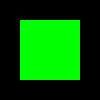initial snapshot
```:
```

Let’s make it a bit more advanced. By keeping track of the widget size, we can provide an array with matching shape. We also take pixel_ratio into account, in case this is a hidpi display, or when the user has used the browser’s zoom.

```:
```
```class HelloWorld2(jupyter_rfb.RemoteFrameBuffer):

def handle_event(self, event):
if event["event_type"] == "resize":
self._size = event
# self.print(event)  # uncomment to display the event

def get_frame(self):
w, h, r = self._size["width"], self._size["height"], self._size["pixel_ratio"]
physical_size = int(h*r), int(w*r)
a = np.zeros((physical_size, physical_size, 3), np.uint8)
margin = int(20 * r)
a[margin:-margin,margin:-margin,1] = 255
return a

w = HelloWorld2()
w
```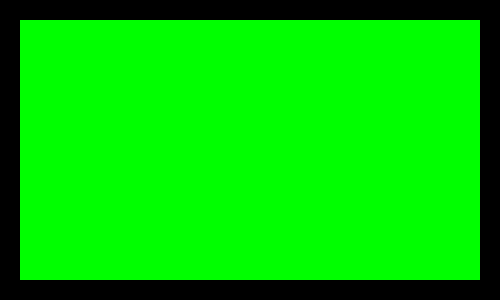initial snapshot
```:
```

If this is a live session, try resizing the widget to see how it adjusts.

```[ ]:
```
```
```

#### Interaction example¶

In this example we implement a simple interaction use-case. This lets you get a feel for the performance (FPS, lag). Note that the snappyness will depend on where the server is (e.g. localhost will work better than MyBinder).

The app presents a dark background with cyan square that can be dragged around.

```:
```
```import numpy as np
import jupyter_rfb
```
```:
```
```class InteractionApp(jupyter_rfb.RemoteFrameBuffer):

def __init__(self):
super().__init__()
self._size = (1, 1, 1)
self._pos = 100, 100
self._drag_pos = None

def handle_event(self, event):
event_type = event.get("event_type", None)
if event_type == "resize":
self._size = event["width"], event["height"], event["pixel_ratio"]
elif event_type == "pointer_down" and event["button"] == 1:
x, y = event["x"], event["y"]
if abs(x - self._pos) < self._radius and abs(y - self._pos) < self._radius:
self._drag_pos = self._pos - x, self._pos - y
self.request_draw()
elif event_type == "pointer_up":
self._drag_pos = None
self.request_draw()
elif event_type == "pointer_move" and self._drag_pos is not None:
self._pos = self._drag_pos + event["x"], self._drag_pos + event["y"]
self.request_draw()

def get_frame(self):
ratio = self._size
w, h = int(self._size * ratio), int(self._size * ratio)
array = np.zeros((h, w, 3), np.uint8)
array[:,:,2] = np.linspace(50, 200, h).reshape(-1, 1)  # bg gradient
array[
1] = 250 if self._drag_pos else 200
return array

w = InteractionApp()
w.max_buffered_frames = 2
w
```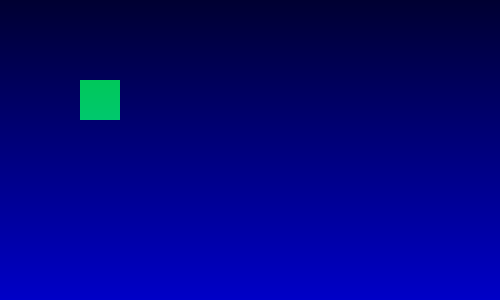initial snapshot
```:
```

You can now interact with the figure by dragging the square to another position.

```:
```
```# Or we can do that programatically :)
w.handle_event({"event_type": "pointer_down", "button": 1, "x": 100, "y": 100})
w.handle_event({"event_type": "pointer_move", "button": 1, "x": 200, "y": 200})
w.handle_event({"event_type": "pointer_up", "button": 1, "x": 200, "y": 200})
```
```:
```
```w.snapshot()
```
```:
```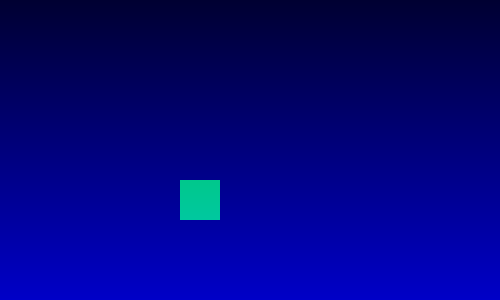snapshot

To get some quantative resuls, run `reset_stats()`, interact, and then call `get_stats()`.

```:
```
```w.reset_stats()
```
```:
```
```w.get_stats()
```
```:
```
```{'sent_frames': 0,
'confirmed_frames': 0,
'roundtrip': 0.0,
'delivery': 0.0,
'img_encoding': 0.0,
'b64_encoding': 0.0,
'fps': 0.0}
```
```[ ]:
```
```
```

#### Interactive drawing example¶

A simple drawing app:

• Draw dots by clicking with LMB.

• Toggle color by clicking RMB.

```:
```
```import numpy as np
import jupyter_rfb
```
```:
```
```class Drawingapp(jupyter_rfb.RemoteFrameBuffer):

def __init__(self):
super().__init__()
self.pixel_ratio = 1 / 8
w, h = 600, 400
self.css_width = f"{w}px"
self.css_height = f"{h}px"
self.resizable = False
self.array = np.ones((int(h*self.pixel_ratio), int(w*self.pixel_ratio), 4), np.uint8) * 5
self.pen_colors = [(1, 0.2, 0, 1), (0, 1, 0.2, 1), (0.2, 0, 1, 1)]
self.pen_index = 0

def handle_event(self, event):
event_type = event.get("event_type", None)
if event_type == "pointer_down":
if event["button"] == 1:
# Draw
x = int(event["x"] * self.pixel_ratio)
y = int(event["y"] * self.pixel_ratio)
self.array[y, x] = 255 * np.array(self.pen_colors[self.pen_index])
self.request_draw()
elif event["button"] == 2:
# Toggle color
self.pen_index = (self.pen_index + 1) % len(self.pen_colors)

def get_frame(self):
return self.array

app = Drawingapp()
app
```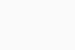initial snapshot
```:
```

After some clicking …

```:
```
```# We can also generate some clicks programatically :)
for x, y in [(503, 37), (27, 182), (182, 383), (396, 235), (477, 151), (328, 308), (281, 16)]:
app.handle_event({"event_type": "pointer_down", "button": 1, "x": x, "y": y})
app.handle_event({"event_type": "pointer_down", "button": 2, "x": 0, "y": 0})
for x, y in [(226, 115), (135, 253), (351, 220), (57, 11), (345, 87), (67, 175), (559, 227)]:
app.handle_event({"event_type": "pointer_down", "button": 1, "x": x, "y": y})
```
```:
```
```app.snapshot()
```
```:
```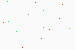snapshot
```:
```
```app.request_draw()
```
```[ ]:
```
```
```

#### Embedding in an ipywidgets app¶

In this example we demonstrate embedding the `RemoteFrameBuffer` class inside a larger ipywidgets app.

```[ ]:
```
```import numpy as np
import ipywidgets
import jupyter_rfb
```

Implement a simple RFB class, for the sake of the example:

```[ ]:
```
```class SimpleRFB(jupyter_rfb.RemoteFrameBuffer):

green_value = 200

def get_frame(self):
a = np.zeros((100, 100, 3), np.uint8)
a[20:-20,20:-20,1] = self.green_value
return a
```

Compose a simple app:

```[ ]:
```
```slider = ipywidgets.IntSlider(min=50, max=255, value=200)
rfb = SimpleRFB()

def on_slider_change(change):
rfb.green_value = change["new"]
rfb.request_draw()

slider.observe(on_slider_change, names='value')
ipywidgets.HBox([rfb, slider])
```
```[ ]:
```
```
```

#### Performance measurements¶

This notebook tries to push a number of frames to the browser as fast as posisble, using different parameters, and measures how fast it goes.

```[ ]:
```
```import time
import numpy as np
from jupyter_rfb import RemoteFrameBuffer

class PerformanceTester(RemoteFrameBuffer):
i = 0
n = 0

def get_frame(self):
if self.i >= self.n:
return None
array = np.zeros((640, 480), np.uint8)
# array = np.random.uniform(0, 255, (640, 480)).astype(np.uint8)
array[:20,: int(array.shape * (self.i + 1) / self.n)] = 255
self.i += 1
self.request_draw()  # keep going
return array

def run(self, n=100):
self.i = 0
self.n = n
self.request_draw()

w = PerformanceTester(css_height='100px')
```
```[ ]:
```
```w
```
```[ ]:
```
```n = 50
w.max_buffered_frames = 2
w.reset_stats()
w.run(n)
```
```[ ]:
```
```# Call this when it's done
w.get_stats()
```
```[ ]:
```
```
```

#### Push example¶

The default behavior of `jupyter_rfb` is to automatically call `get_frame()` when a new draw is requested and when the widget is ready for it. In use-cases where you want to push frames to the widget, you may prefer a different approach. Here is an example solution.

```[ ]:
```
```import numpy as np
from jupyter_rfb import RemoteFrameBuffer

class FramePusher(RemoteFrameBuffer):
def __init__(self, **kwargs):
super().__init__(**kwargs)
self._queue = []

def push_frame(self, frame):
self._queue.append(frame)
self._queue[:-10] = [] # drop older frames if len > 10
self.request_draw()

def get_frame(self):
if not self._queue:
return
self.request_draw()
return self._queue.pop(0)

w = FramePusher(css_width='100px', css_height='100px')
w
```
```[ ]:
```
```w.push_frame(np.random.uniform(0, 255, (100, 100)).astype(np.uint8))
```
```[ ]:
```
```# Push 20 frames. Note that only the latest 10 will be shown
for i in range(20):
w.push_frame(np.random.uniform(0, 255, (100, 100)).astype(np.uint8))
len(w._queue)
```
```[ ]:
```
```
```

#### PyGfx cube example¶

Note that this example depends on pygfx (``pip install -U pygfx``).

An example showing a lit, textured, rotating cube.

```:
```
```import numpy as np
import imageio
import pygfx as gfx

from wgpu.gui.jupyter import WgpuCanvas

canvas = WgpuCanvas()
renderer = gfx.renderers.WgpuRenderer(canvas)
scene = gfx.Scene()

geometry = gfx.BoxGeometry(200, 200, 200)
geometry.texcoords.data[:] *= 2  # smaller bricks
material = gfx.MeshPhongMaterial(map=tex, color=(1, 0, 0, 0.2), clim=(0.2, 0.8))
cube = gfx.Mesh(geometry, material)

camera = gfx.PerspectiveCamera(70, 16 / 9)
camera.position.z = 400

def animate():
rot = gfx.linalg.Quaternion().set_from_euler(gfx.linalg.Euler(0.005, 0.01))
cube.rotation.multiply(rot)

renderer.render(scene, camera)
canvas.request_draw()

canvas.request_draw(animate)
canvas
```
```Forcing backend: Vulkan (4)
```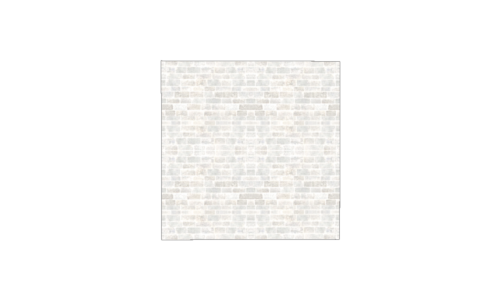initial snapshot
```:
```
```[ ]:
```
```
```

#### PyGfx points and lines example¶

Note that this example depends on pygfx (``pip install -U pygfx``).

An example demonstrating points and lines, in two separate canvases.

```:
```
```import numpy as np
import pygfx as gfx
from wgpu.gui.jupyter import WgpuCanvas
```
```:
```
```canvas1 = WgpuCanvas()
renderer1 = gfx.WgpuRenderer(canvas1)

scene1 = gfx.Scene()

x = np.linspace(20, 620, 200, dtype=np.float32)
y = np.sin(x / 10) * 100 + 200

positions = np.column_stack([x, y, np.zeros_like(x)])
colors = np.random.uniform(0, 1, (x.size, 4)).astype(np.float32)
geometry = gfx.Geometry(positions=positions, colors=colors)

# Also see LineSegmentMaterial and LineThinSegmentMaterial
material = gfx.LineSegmentMaterial(
thickness=6.0, color=(0.0, 0.7, 0.3, 0.5), vertex_colors=True
)
line = gfx.Line(geometry, material)

camera1 = gfx.ScreenCoordsCamera()

canvas1.request_draw(lambda: renderer1.render(scene1, camera1))
canvas1
```
```Forcing backend: Vulkan (4)
```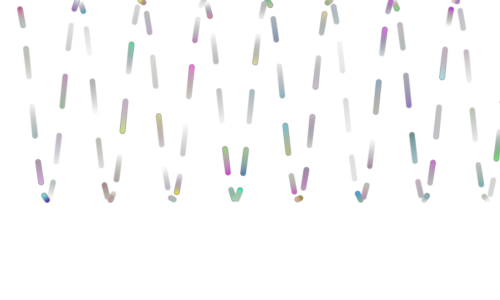initial snapshot
```:
```
```:
```
```canvas2 = WgpuCanvas()
renderer2 = gfx.WgpuRenderer(canvas2)

scene2 = gfx.Scene()

positions = np.random.normal(0, 0.5, (100, 3)).astype(np.float32)
geometry = gfx.Geometry(positions=positions)

material = gfx.PointsMaterial(size=10, color=(0, 1, 0.5, 0.7))
points = gfx.Points(geometry, material)
scene2.add(gfx.Background(gfx.BackgroundMaterial((0.2, 0.0, 0, 1), (0, 0.0, 0.2, 1))))

camera2 = gfx.NDCCamera()

canvas2.request_draw(lambda: renderer2.render(scene2, camera2))
canvas2
```
```Forcing backend: Vulkan (4)
```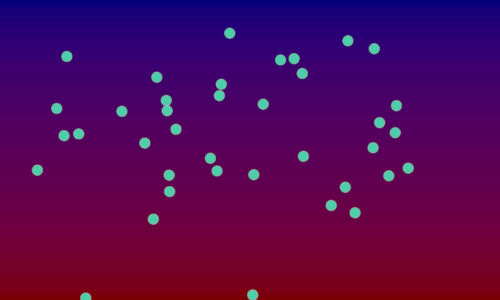initial snapshot
```:
```
```[ ]:
```
```
```

#### PyGfx picking example¶

Note that this example depends on pygfx (``pip install -U pygfx``).

An example demonstrating pickable points.

```:
```
```import numpy as np
import pygfx as gfx
from wgpu.gui.jupyter import WgpuCanvas

class PickingWgpuCanvas(WgpuCanvas):
def handle_event(self, event):
super().handle_event(event)
# Get a dict with info about the clicked location
if event["event_type"] == "pointer_down":
xy = event["x"], event["y"]
info = renderer.get_pick_info(xy)
wobject = info["world_object"]
# If a point was clicked ..
if wobject and "vertex_index" in info:
i = int(round(info["vertex_index"]))
geometry.positions.data[i, 1] *= -1
geometry.positions.update_range(i)
canvas.request_draw()

canvas = PickingWgpuCanvas()
renderer = gfx.renderers.WgpuRenderer(canvas)
scene = gfx.Scene()

xx = np.linspace(-50, 50, 10)
yy = np.random.uniform(20, 50, 10)
geometry = gfx.Geometry(positions=[(x, y, 0) for x, y in zip(xx, yy)])
if True:  # Set to False to try this for a line
ob = gfx.Points(geometry, gfx.PointsMaterial(color=(0, 1, 1, 1), size=16))
else:
ob = gfx.Line(geometry, gfx.LineMaterial(color=(0, 1, 1, 1), thickness=10))

camera = gfx.OrthographicCamera(120, 120)

canvas.request_draw(lambda: renderer.render(scene, camera))
canvas
```
```Forcing backend: Vulkan (4)
```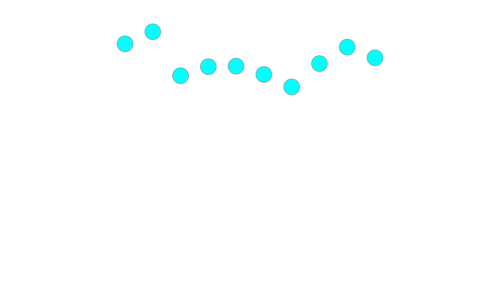initial snapshot
```:
```
```[ ]:
```
```
```

#### Video example¶

This example depends on imageio and imageio-ffmpeg.

Plays a video as fast as it can. This will be quite a slow playback on localhost. On a remote server it will be even slower.

```:
```
```import imageio
import numpy as np
from jupyter_rfb import RemoteFrameBuffer

class VideoPlayer(RemoteFrameBuffer):

def get_frame(self):
v.request_draw()
return r.get_next_data()

v = VideoPlayer(max_buffered_frames=3)
v
```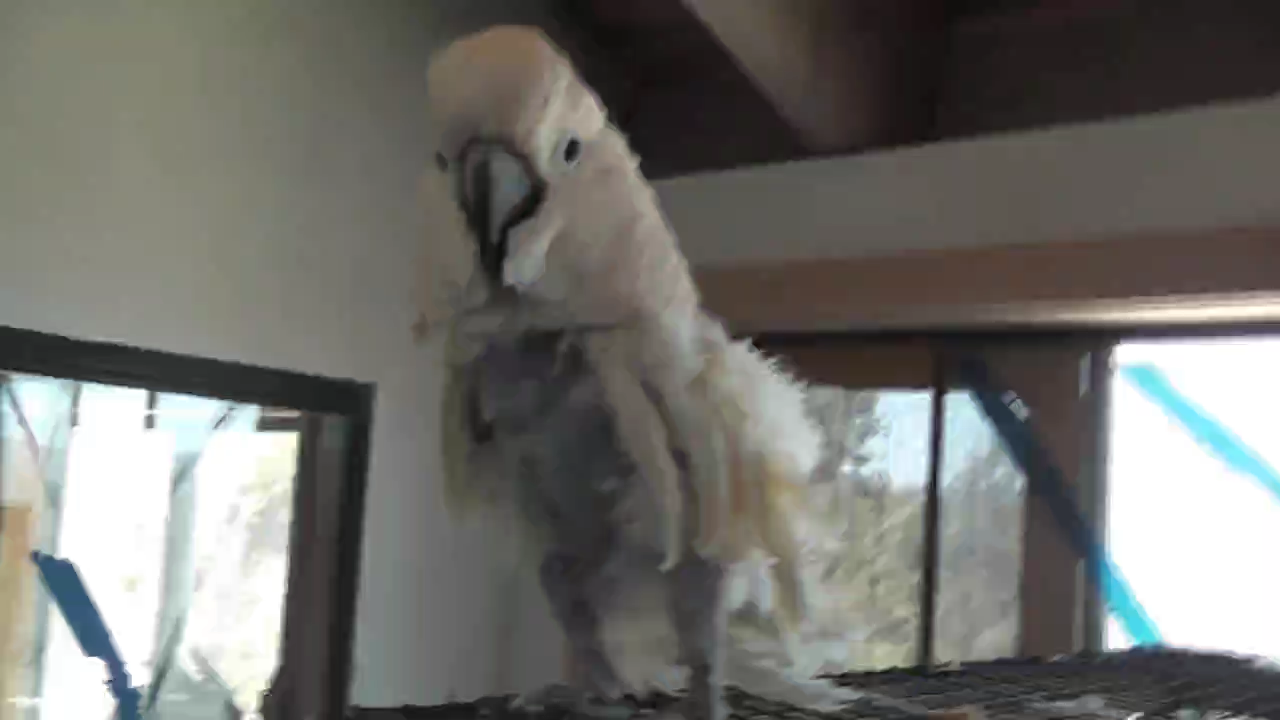initial snapshot
```:
```
```[ ]:
```
```
```

#### Visibility check¶

This notebook is to verify that widgets that are not visible do not perform any draws.

```[ ]:
```
```import asyncio
import numpy as np
from jupyter_rfb import RemoteFrameBuffer
```
```[ ]:
```
```class ProgressBar(RemoteFrameBuffer):

i = 0
n = 32
channel = 0
callback = lambda *args: None

def get_frame(self):
self.callback()
self.i += 1
if self.i >= self.n:
self.i = 0
array = np.zeros((100, 600, 3), np.uint8)
array[:,: int(array.shape * (self.i + 1) / self.n), se```